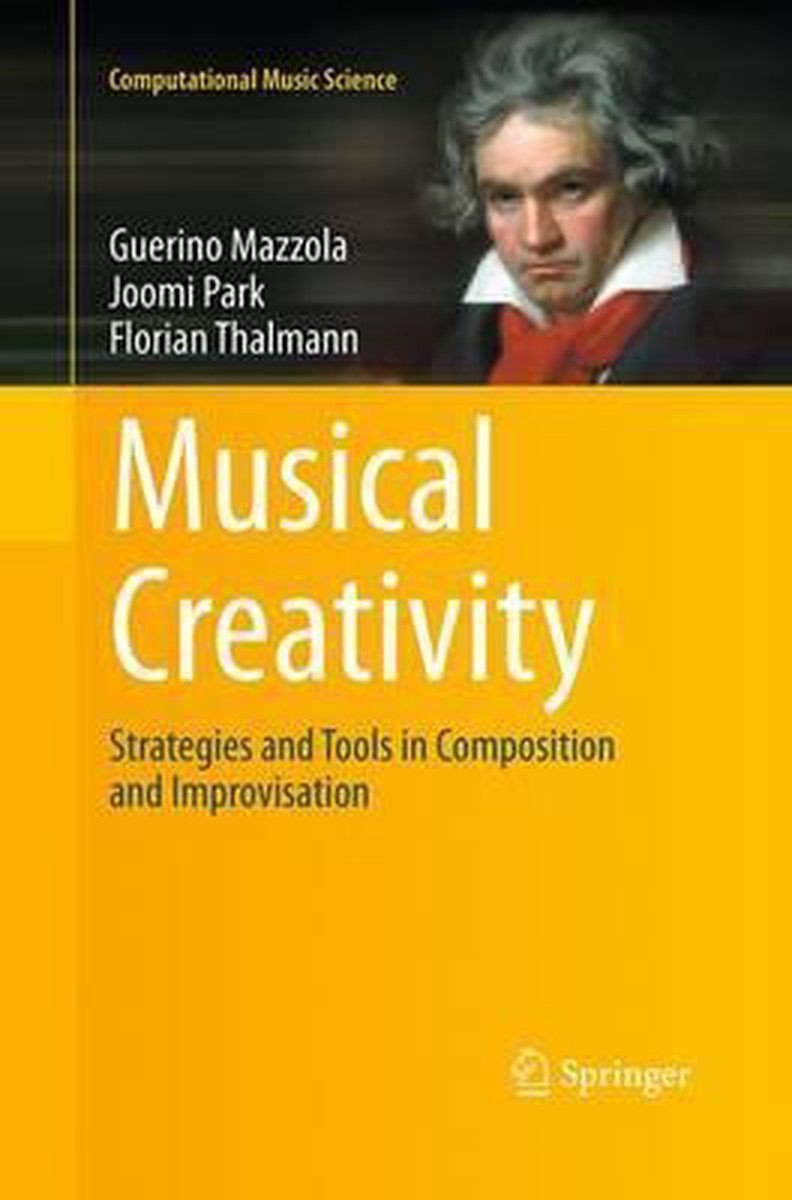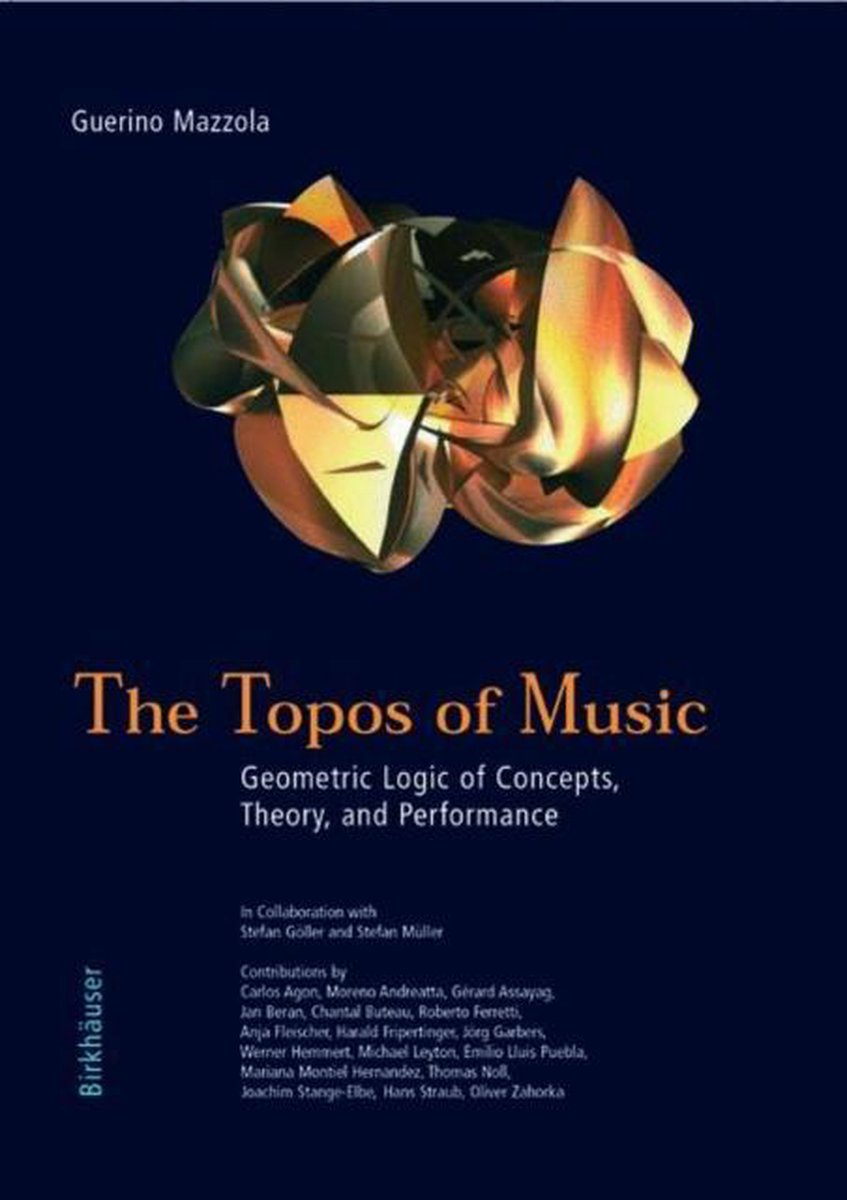# Tag: condensed math

In a recent post I recalled Claude Levy-Strauss’ observation “In Paris, intellectuals need a new toy every 15 years”, and gave a couple of links showing that the most recent IHES-toy has been spreading to other Parisian intellectual circles in recent years.

At the time (late sixties), Levy-Strauss was criticising the ongoing Foucault-hype. It appears that, since then, the frequency of a hype cycle is getting substantially shorter.

Ten days ago, the IHES announced that Dustin Clausen (of condensed math fame) is now joining the IHES as a permanent professor.

To me, this seems like a sensible decision, moving away from (too?) general topos theory towards explicit examples having potential applications to arithmetic geometry.

On the relation between condensed sets and toposes, here’s Dustin Clausen talking about “Toposes generated by compact projectives, and the example of condensed sets”, at the “Toposes online” conference, organised by Alain Connes, Olivia Caramello and Laurent Lafforgue in 2021.

Two days ago, Clausen gave another interesting (inaugural?) talk at the IHES on “A Conjectural Reciprocity Law for Realizations of Motives”.

Some months ago, Peter Scholze wrote a guest post on the Xena-blog: Liquid tensor experiment, proposing a challenge to formalise the proof of one of his results with Dustin Clausen on condensed mathematics.

Scholze and Clausen ran a masterclass in Copenhagen on condensed mathematics, which you can binge watch on YouTube starting here

Scholze also gave two courses on the material in Bonn of which the notes are available here and here.

Condensed mathematics claims that topological spaces are the wrong definition, and that one should replace them with the slightly different notion of condensed sets.

So, let’s find out what a condensed set is.

Definition: Condensed sets are sheaves (of sets) on the pro-étale site of a point.

(there’s no danger we’ll have to rewrite our undergraduate topology courses just yet…)

In his blogpost, Scholze motivates this paradigm shift by observing that the category of topological Abelian groups is not Abelian (if you put a finer topology on the same group then the identity map is not an isomorphism but doesn’t have a kernel nor cokernel) whereas the category of condensed Abelian groups is.

It was another Clausen-Scholze result in the blogpost that caught my eye.

But first, for something completely different.

In “Musical creativity”, Guerino Mazzola and co-authors introduce a seven steps path to creativity.Here they are:

1. Exhibiting the open question
2. Identifying the semiotic context
3. Finding the question’s critical sign
4. Identifying the concept’s walls
5. Opening the walls
6. Displaying extended wall perspectives
7. Evaluating the extended walls

Looks like a recipe from distant flower-power pot-infused times, no?

In Towards a Categorical Theory of Creativity for Music, Discourse, and Cognition, Mazzola, Andrée Ehresmann and co-authors relate these seven steps to the Yoneda lemma.

1. Exhibiting the open question = to understand the object $A$
2. Identifying the semiotic context = to describe the category $\mathbf{C}$ of which $A$ is an object
3. Finding the question’s critical sign = $A$ (?!)
4. Identifying the concept’s walls = the uncontrolled behaviour of the Yoneda functor
$@A~:~\mathbf{C} \rightarrow \mathbf{Sets} \qquad C \mapsto Hom_{\mathbf{C}}(C,A)$
5. Opening the walls = finding an objectively creative subcategory $\mathbf{A}$ of $\mathbf{C}$
6. Displaying extended wall perspectives = calculate the colimit $C$ of a creative diagram
7. Evaluating the extended walls = try to understand $A$ via the isomorphism $C \simeq A$.

(Actually, I first read about these seven categorical steps in another paper which might put a smile on your face: The Yoneda path to the Buddhist monk blend.)

Remains to know what a ‘creative’ subcategory is.

The creative moment comes in here: could we not find a subcategory
$\mathbf{A}$ of $\mathbf{C}$ such that the functor
$Yon|_{\mathbf{A}}~:~\mathbf{C} \rightarrow \mathbf{PSh}(\mathbf{A}) \qquad A \mapsto @A|_{\mathbf{A}}$
is still fully faithful? We call such a subcategory creative, and it is a major task in category theory to find creative categories which are as small as possible.

All the ingredients are here, but I had to read Peter Scholze’s blogpost before the penny dropped.

Let’s try to view condensed sets as the result of a creative process.

1. Exhibiting the open question: you are a topologist and want to understand a particular compact Hausdorff space $X$.
2. Identifying the semiotic context: you are familiar with working in the category $\mathbf{Tops}$ of all topological spaces with continuous maps as morphisms.
3. Finding the question’s critical sign: you want to know what differentiates your space $X$ from all other topological spaces.
4. Identifying the concept’s walls: you can probe your space $X$ with continuous maps from other topological spaces. That is, you can consider the contravariant functor (or presheaf on $\mathbf{Tops}$)
$@X~:~\mathbf{Tops} \rightarrow \mathbf{Sets} \qquad Y \mapsto Cont(Y,X)$
and Yoneda tells you that this functor, up to equivalence, determines the space $X$ upto homeomorphism.
5. Opening the walls: Tychonoff tells you that among all compact Hausdorff spaces there’s a class of pretty weird examples: inverse limits of finite sets (or a bit pompous: the pro-etale site of a point). These limits form a subcategory $\mathbf{ProF}$ of $\mathbf{Tops}$.
6. Displaying extended wall perspectives: for every inverse limit $F \in \mathbf{ProF}$ (for ‘pro-finite sets’) you can look at the set $\mathbf{X}(F)=Cont(F,X)$ of all continuous maps from $F$ to $X$ (that is, all probes of $X$ by $F$) and this functor
$\mathbf{X}=@X|_{\mathbf{ProF}}~:~\mathbf{ProF} \rightarrow \mathbf{Sets} \qquad F \mapsto \mathbf{X}(F)$
is a sheaf on the pre-etale site of a point, that is, $\mathbf{X}$ is the condensed set associated to $X$.
7. Evaluating the extended walls: Clausen and Scholze observe that the assignment $X \mapsto \mathbf{X}$ embeds compact Hausdorff spaces fully faithful into condensed sets, so we can recover $X$ up to homeomorphism as a colimit from the condenset set $\mathbf{X}$. Or, in Mazzola’s terminology: $\mathbf{ProF}$ is a creative subcategory of $\mathbf{(cH)Tops}$ (all compact Hausdorff spaces).

It would be nice if someone would come up with a new notion for me to understand Mazzola’s other opus “The topos of music” (now reprinted as a four volume series).